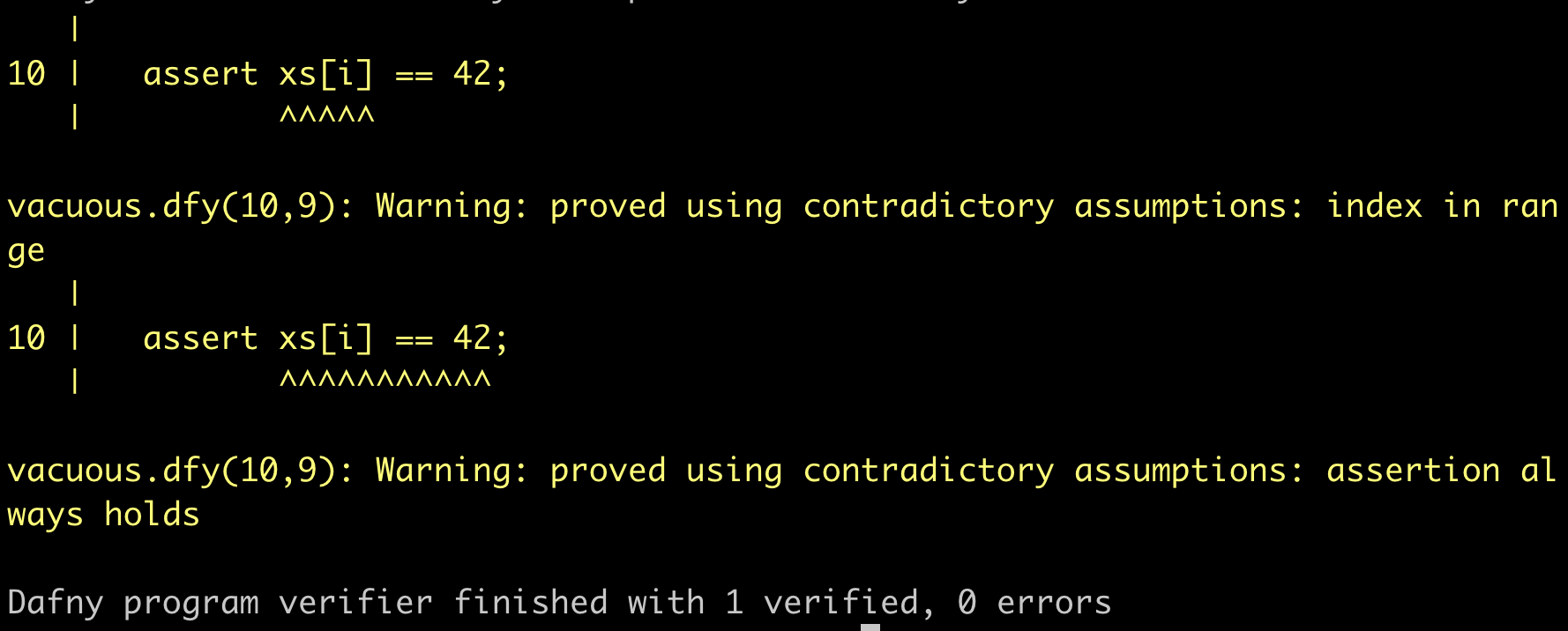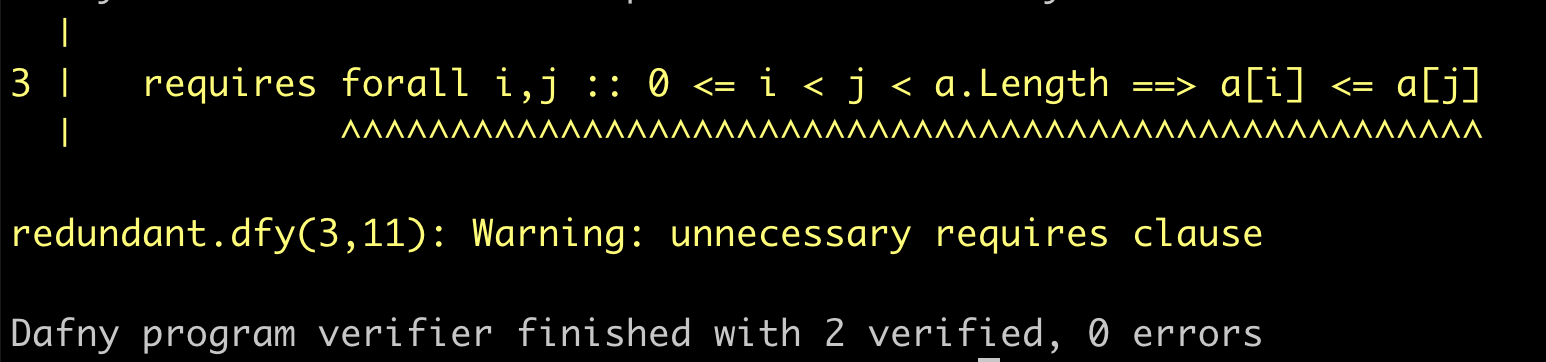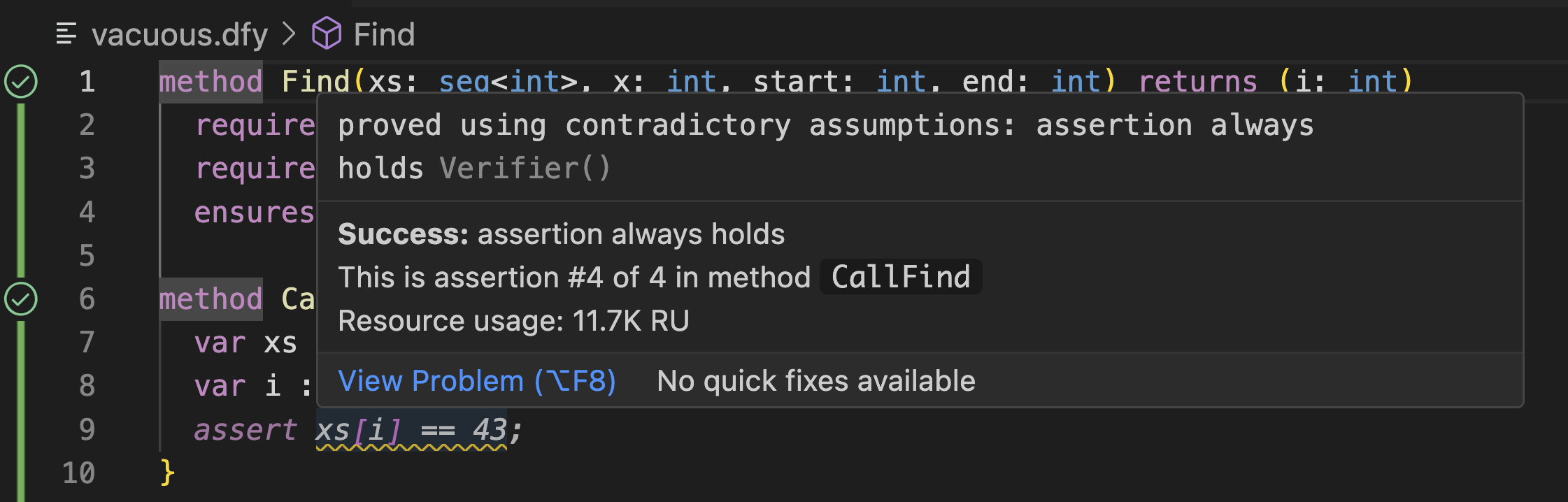## Introduction

Problems in a program's specification can undermine the trust obtained from verification. If the specification doesn't actually state what you intended it to state, the verification may still be technically sound but provide a false sense of security that the program behaves as intended. There are many ways that the text of a specification may differ from its intent, but there are some specific signs of potential mistakes that can be stated formally and reasoned about automatically.

For example, if the assumptions you make in your specification are contradictory, any program will satisfy it. Contradictory specifications are particularly easy to construct by accident when writing assumed specifications about external or yet-to-be-written code. To see what a contradictory specification might look like, consider the following code with an unimplemented declaration of Find, to find an element in a sequence, and an implemented caller. This sort of situation might occur during the development process, as you experiment with how you want to structure your program.

  method Find(xs: seq<int>, x: int, start: int, end: int) returns (i: int)
requires start < |xs|
requires end < |xs|
ensures start < i < end

method CallFind() {
var xs := [42, 43];
var i := Find(xs, 43, 0, 1);
assert xs[i] == 42;
}

Here, the Find method has a roughly reasonable-looking (if incomplete) specification. However, it turns out that the ensures clause is false if start and end are the same or adjacent indices, and CallFind passes in adjacent indices. Therefore, it's possible to prove a false assertion after the call.

As another example, a program may contain assumptions, or intermediate assertions, that are unnecessary for constructing a proof of correctness. These redundant specifications may indicate a mistake, such that they would not be redundant if written correctly. Or it may be possible to remove them, simplifying the program and proof process. In the following example, the precondition (which one might think would be required to make the code well-defined) turns out to be unnecessary. Why it's unnecessary is left to you as a thought exercise: do we really need the array to be sorted to ensure that the array indices are always in bounds?

  method BinarySearchRedundant(a: array<int>, key: int) returns (r: int)
requires forall i,j :: 0 <= i < j < a.Length ==> a[i] <= a[j]
{
var lo, hi := 0, a.Length;
while lo < hi
invariant 0 <= lo <= hi <= a.Length
{
var mid := (lo + hi) / 2;
if key < a[mid] {
hi := mid;
} else if a[mid] < key {
lo := mid + 1;
} else {
return mid;
}
}
return -1;
}

As a final example, if the specification doesn't cover all of the program's behavior, any implementation will satisfy the uncovered portions. This latter case is frequently intentional you may only want to prove certain simple properties about your program, such as the lack of runtime errors but it can be useful to confirm that the unspecified portions do not include code you intended to specify.

In the following example of a binary search implementation, we removed the redundant assumption, and Dafny verifies only the absence of undefined behavior. In particular, it doesn't prove that the return value correctly corresponds to what you'd expect from a binary search implementation. Therefore, any of the return statements could be modified without causing a verification failure.

  method BinarySearch(a: array<int>, key: int) returns (r: int)
{
var lo, hi := 0, a.Length;
while lo < hi
invariant 0 <= lo <= hi <= a.Length
{
var mid := (lo + hi) / 2;
if key < a[mid] {
hi := mid;
} else if a[mid] < key {
lo := mid + 1;
} else {
return mid; // Unconstrained by specification
}
}
return -1; // Unconstrained by specification
}

## Proof dependencies in Dafny

With v4.4.01 Dafny will include features to automatically detect problems like contradictory specifications, redundant assumptions, and unspecified code, using a notion of proof dependency. A proof dependency is a portion of a program that needed to be taken into account in order to construct a proof that the program is correct. When talking about proof dependencies, let's use the term property more formally from here on to refer a particular statement about a Dafny program that we want to prove. This might arise from an assert statement, an ensures clause, or a requires clause of a call, for example. Let's use assumption to refer to anything that's assumed, arising, for example, from an assume statement, a requires clause, or an ensures clause of a call.

Proof dependencies make it possible to identify properties proved due to contradictory assumptions. To legitimately prove that a property holds of the program, it must be the case that the proof takes the property itself into account. If it's possible to construct a proof that the property holds without considering what it is we're ultimately trying to prove, we say that we've proved it vacuously. This can occur because the assumptions in scope at that program location are contradictory, or that that location is unreachable.

Similarly, any assumptions that never appear in the dependencies of the proof of any property can be removed. This also applies to the assumptions that arise from the use of intermediate assert statements that exist as stepping stones toward proving a later property. If they weren't actually used to prove any later property, they can be removed.

Finally, proof dependencies can identify unspecified portions of an implementation. If it's possible to prove a program correct without taking into account certain implementation statements, then those statements are unconstrained by the verification. They could be changed arbitrarily without causing verification to fail.

Three new flags to the dafny verify command enable proof dependency calculation.

The --warn-contradictory-assumptions flag instructs Dafny to emit a warning any time it completes a proof without taking the goal into account, indicating a dependence on contradictory assumptions. For example, it produces the following output on the CallFind method above that calls an incorrectly-specified (and unimplemented) Find method.The --warn-redundant-assumptions flag instructs Dafny to emit a warning any time an assumption in scope (from an assume statement or requires clause) was not required to complete any proof goal. On the binary search example above, with an unnecessary requires clause, Dafny produces the following.These options can be enabled in the IDE, as well. We recommend doing this in a dfyconfig.toml file such as the following:

[options]
warn-redundant-assumptions = true
warn-contradictory-assumptions = true

With this in place, the example of contradictory assumptions shown above looks something like the following in Visual Studio Code:To identify portions of a program that were not included in verification currently requires a slightly more manual step. Consider the unspecified version of binary search again.

  method BinarySearch(a: array<int>, key: int) returns (r: int)
{
var lo, hi := 0, a.Length;
while lo < hi
invariant 0 <= lo <= hi <= a.Length
{
var mid := (lo + hi) / 2;
if key < a[mid] {
hi := mid;
} else if a[mid] < key {
lo := mid + 1;
} else {
return mid; // Unconstrained by specification
}
}
return -1; // Unconstrained by specification
}

Dafny includes a verification logger that will describe the status of and statistics about each verification goal in the program. When proof dependency analysis is enabled, this will include information about which potential proof dependencies did or did not take place in the actual proof. This information will only be included if one of the other flags enabling proof dependency analysis is enabled, however. So, if we analyze the binary search example using the command dafny verify --log-format text --warn-redundant-assertions, the output will include the following text.

    Unused by proof:
unspecified.dfy(4,14)-(4,28): assignment (or return)
unspecified.dfy(4,14)-(4,28): assignment (or return)
unspecified.dfy(6,15)-(6,34): loop invariant
unspecified.dfy(10,7)-(10,16): assignment (or return)
unspecified.dfy(14,7)-(14,17): assignment (or return)
unspecified.dfy(17,3)-(17,12): assignment (or return)
unspecified.dfy(18,1)-(18,1): out-parameter 'r', which is subject to definite-assignment rules, is always initialized at this return point

This shows that the assignment/return statements on lines 10, 14, and 17, for example, were not necessary to show that the method is well-defined.

Enabling proof dependency calculation requires additional work as part of the proof process. This means it can slow down verification, and potentially cause especially brittle proofs to fail. In our experiments so far, it can add ~30% to verification time. If your project is developed with the feature turned on from the start, however, this cost can be mitigated, especially when taking verification optimization guidance into account.

## Theory and implementation

Internally, Dafny's proof dependency analysis is built on the common SMT feature of unsatisfiable cores. Dafny encodes each verification goal as an SMT query that negates the original goal. This means that a conclusion that the negated goal is unsatisfiable (i.e., no value exists that will make it true) means that the original goal is valid (i.e., true for all possible values). Many SMT solvers, including the Z3 solver that Dafny uses by default, can accompany a conclusion of “unsatisfiable” with a subset of the sub-expressions (clauses, in SMT terminology) from the original goal that is still unsatisfiable. This subset is generally smaller than the original formula, though it is not guaranteed to be so, and is not guaranteed to be minimal.

Because unsatisfiable cores are not guaranteed to be minimal, Dafny may sometimes fail to warn about some goals that are proved using a contradiction, or some assumptions that are not ultimately necessary. In our early experience, however, Dafny does produce useful warnings for all large code bases we've tried it on.

## Summary

Dafny can now integrate proof dependency analysis into the verification process, enabling it to warn about contradictory and redundant assumptions and enabling you to identify unspecified code. We encourage you to turn on the new warnings in your code as a standard part of your verification workflow, ideally using the dfyconfig.toml entries listed above. Although this analysis incurs some performance overhead, the extra computational work provides higher trust that you've verified what you really intended.

1.Or any nightly build after 2023-10-24.